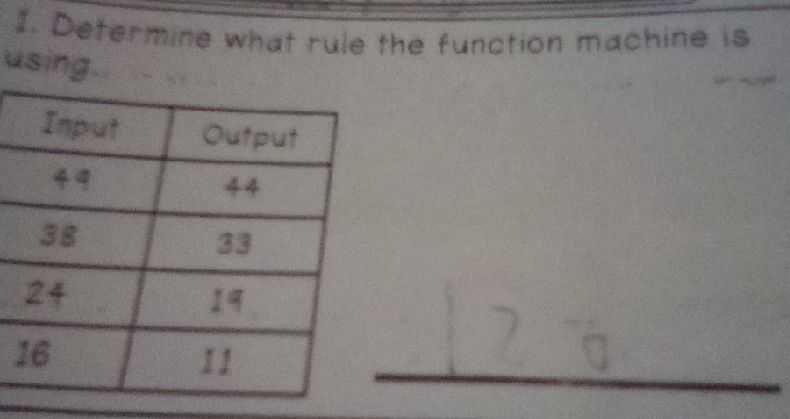### ¿Todavía tienes preguntas de matemáticas?

Pregunte a nuestros tutores expertos
Algebra
Pregunta7. Determine what rule the function machine is using.

Input           Output

$$49$$               $$44$$

$$38$$               $$33$$

$$24$$               $$19$$

$$16$$               $$11$$

Your input: find the equation of a line given two points    $$P = ( 49,44 )$$ and $$Q = ( 38,33 )$$ . The slope of a line passing through the two points $$P = ( x _ { 1 } , y _ { 1 } )$$ and $$Q = ( x _ { 2 } , y _ { 2 } )$$ is given by $$m = \frac { y _ { 2 } - y _ { 1 } } { x _ { 2 } - x _ { 1 } }$$

We have that $$x _ { 1 } = 49 , y _ { 1 } = 44 , x _ { 2 } = 38 , y _ { 2 } = 33$$

Plug the given values into the formula for slope: $$m = \frac { ( 33 ) - ( 44 ) } { ( 38 ) - ( 49 ) } = \frac { - 11 } { - 11 } = 1$$

Now, the $$y$$ -intercept is $$b = y _ { 1 } - m \cdot x _ { 1 }$$ (or $$b = y _ { 2 } - m \cdot x _ { 2 }$$ , the result is the same).

$$b = 44 - ( 1 ) \cdot ( 49 ) = - 5$$ .

Finally, the equation of the line can be written in the form $$y = m x + b$$ .

$$y = x - 5$$

The slope of the line is $$m = 1$$
The equation of the line in the slope-intercept form is $$y = x - 5$$ .
The equation of the line in the point-slope form is $$y - 44 = 1 ( x - 49 )$$ .
The equation of the line in the point-slope form is $$y - 33 = 1 ( x - 38 )$$ .
The general equation of the line is $$x - y - 5 = 0$$ .